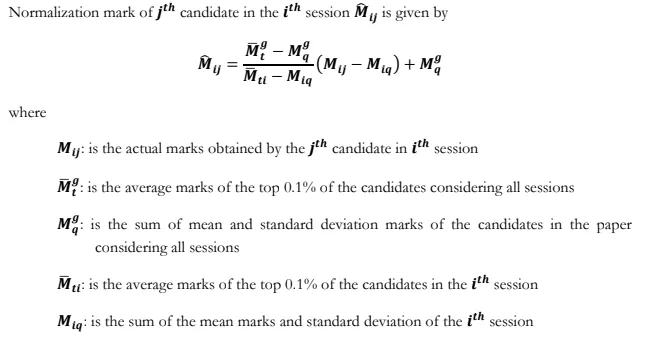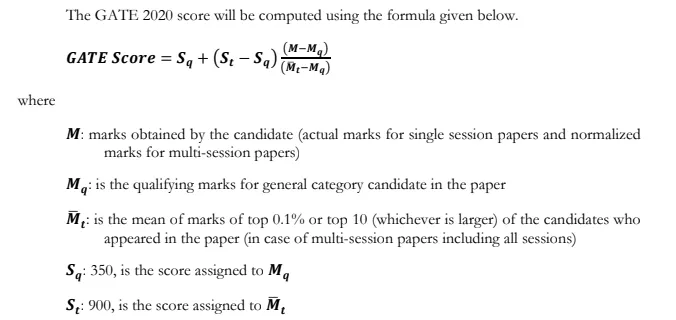Engineering Jobs   »   GATE 2023   »   GATE 2023 Normalization

# GATE 2023 Normalization, Formula, How GATE Score Calculated?

## GATE 2023 Normalization

IIT Kanpur has organized the GATE 2023 Exam on the 4th, 5th, 11th, and 12th of February 2023 for 29 different disciplines. Some GATE Papers were conducted in more than one session like Mechanical, Computer Science, Civil, and Electrical. The question papers held in multiple session has a possibility to vary their difficulty level.

To equate the difficulty level of the exam, conducting authority employed a normalization process. We have discussed the GATE 2023 Normalization Process in detail here for the ease of aspirants. So, refer to this article to understand the GATE 2023 Normalization and bookmark this site for the latest Engineering Updates.

## GATE 2023 Normalization

GATE 2023 Normalization is a technique to determine the score of candidates equal by considering the difficulty level of all session papers. The GATE Score of candidates is determined on a scale of 1000 using a specified formula. The score of aspirants comes out of 1000 is considered as their GATE Score. Most of the aspirants are confused about how their score is calculated and how the normalization procedure is employed. Check a detailed discussion about GATE 2023 Normalization summarized in this post.

Check: GATE 2024 Details

## What is Normalization in GATE?

Some of the papers in GATE 2023 were conducted in multiple shifts. Due to the multiple session, the difficulty level of the GATE question paper varies. In order to calculate a fair score, the conducting institute made a normalization process. The students who appeared in the GATE Exam might be thinking about how the normalization procedure is employed to decide the score of candidate. Check the normalization procedure steps, normalization formula, etc.

UGC NET vs GATE

## GATE Marks vs Score

The GATE Score of candidates is calculated out of 1000 while the marks of candidates will be evaluated out of 100 points. The result of the GATE Exam has been declared with the score and marks. The score of candidates is decided by applying the normalization formula. GATE Score card is released only for the qualified candidates whose score will be above than the cutoff announced by the authority.

The GATE Score Card is used for various purposes like admission to M. Tech/MS/Ph.D. program and also for PSU Recruitments. The validity of the GATE Score is 3 years for admission and recruitment purposes.

Check: Benefits of GATE Exam

## How GATE Score is Calculated?

The GATE Score is calculated by demonstrating a formula out of 1000. The marks secured by candidates out of 100 are converted to scores on a scale of 1000. The normalization formula is used to equate the variation in the difficulty levels of the question papers conducted in different sessions. To set the GATE Score, a normalization formula is used shown below in the article.

## GATE Normalization Formula

The normalization formula is applied to calculate the GATE Score based on the standard deviation and variance. Check the GATE Normalization Formula below to briefly understand the normalization procedure.## GATE Score Calculation Formula

For the GATE Paper held in a single session, the actual marks obtained by the candidates are used for calculating the GATE Score. But for the papers conducted in multi-sessions, normalized marks are used for calculating the GATE score. The formula used for the calculation of the GATE Score is given below.## GATE Exam Result

The GATE Exam Result was out on 16th March 2023. The IIT Kanpur declared the GATE Result on its official portal @gate.iitk.ac.in. The aspirants who appeared in the GATE Exam conducted on the 4th, 5th, 11th, and 12th of February 2023 can check their results using the link provided below. To check the GATE 2023 Exam Result, candidates must have an Enrollment ID/Email ID and Password. Follow the link available here to redirect to the result page of GATE without visiting the official website.

## GATE Score Card 2023

The GATE Score Card of the qualified candidates has been released on 21st March 2023 on the official portal of GATE 2023 @gate.iitk.ac.in.  The GATE Score will be valid for 3 years which is considered for admission to PG Programs in the ME/M. Tech/MS/Ph.D. courses in the IITs or other reputed institutions and it is also accepted to offer PSU Jobs. The candidates can download their GATE Score Card 2023 using the link provided below.

Sharing is caring!

## FAQs

### What is the purpose of GATE 2023 Normalization?

The GATE 2023 Normalization is employed to set the unbiased GATE Score for the papers conducted in multiple shifts.

### What is the formula for GATE 2023 Normalization?

The GATE 2023 Normalization Formula is given in the article.

### When will the GATE Result declared?

The GATE Result will be declared on 16th March 2023.

### How can I check my GATE 2023 Score?

Candidates will be able to check their GATE 2023 Score after the declaration of the result.

### How GATE Score is calculated out of 1000?

The marks of candidates in the GATE Exam are converted on a scale of 1000 by multiplying it by 10. For example, if the candidate's marks are 60 then his GATE Score will be 60*10 = 600.

### TOPICS:

[related_posts_view]